Press "Enter" to skip to content

# Posts published in “Graph”

Given the head of a graph, return a deep copy (clone) of the graph. Each node in the graph contains a label (int) and a list (List[UndirectedGraphNode]) of its neighbors. There is an edge between the given node and each of the nodes in its neighbors.
OJ’s undirected graph serialization (so you can understand error output):

Nodes are labeled uniquely.We use # as a separator for each node, and , as a separator for node label and each neighbor of the node.

As an example, consider the serialized graph {0,1,2#1,2#2,2}.

The graph has a total of three nodes, and therefore contains three parts as separated by #.

1. First node is labeled as 0. Connect node 0 to both nodes 1 and 2.
2. Second node is labeled as 1. Connect node 1 to node 2.
3. Third node is labeled as 2. Connect node 2 to node 2 (itself), thus forming a self-cycle.

Visually, the graph looks like the following:

       1
/ \
/   \
0 --- 2
/ \
\_/


Note: The information about the tree serialization is only meant so that you can understand error output if you get a wrong answer. You don’t need to understand the serialization to solve the problem.

## Solution: Queue + Hashtable

Time complexity: O(V+E)
Space complexity: O(V+E)

## C++

Given an array equations of strings that represent relationships between variables, each string equations[i] has length 4 and takes one of two different forms: "a==b" or "a!=b".  Here, a and b are lowercase letters (not necessarily different) that represent one-letter variable names.

Return true if and only if it is possible to assign integers to variable names so as to satisfy all the given equations.

Example 1:

Input: ["a==b","b!=a"]
Output: false
Explanation: If we assign say, a = 1 and b = 1, then the first equation is satisfied, but not the second.  There is no way to assign the variables to satisfy both equations.


Example 2:

Input: ["b==a","a==b"]
Output: true
Explanation: We could assign a = 1 and b = 1 to satisfy both equations.


Example 3:

Input: ["a==b","b==c","a==c"]
Output: true


Example 4:

Input: ["a==b","b!=c","c==a"]
Output: false


Example 5:

Input: ["c==c","b==d","x!=z"]
Output: true


Note:

1. 1 <= equations.length <= 500
2. equations[i].length == 4
3. equations[i] and equations[i] are lowercase letters
4. equations[i] is either '=' or '!'
5. equations[i] is '='

## Solution: Union Find

Time complexity: O(n)
Space complexity: O(1)

## C++

In a N x N grid composed of 1 x 1 squares, each 1 x 1 square consists of a /\, or blank space.  These characters divide the square into contiguous regions.

(Note that backslash characters are escaped, so a \ is represented as "\\".)

Return the number of regions.

Example 1:

Input:[  " /",  "/ "]Output: 2Explanation: The 2x2 grid is as follows:Example 2:

Input:[  " /",  "  "]Output: 1Explanation: The 2x2 grid is as follows: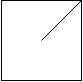Example 3:

Input:[  "\/",  "/\"]Output: 4Explanation: (Recall that because \ characters are escaped, "\/" refers to \/, and "/\" refers to /\.)The 2x2 grid is as follows: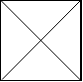Example 4:

Input:[  "/\",  "\/"]Output: 5Explanation: (Recall that because \ characters are escaped, "/\" refers to /\, and "\/" refers to \/.)The 2x2 grid is as follows: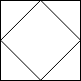Example 5:

Input:[  "//",  "/ "]Output: 3Explanation: The 2x2 grid is as follows: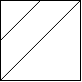Note:

1. 1 <= grid.length == grid.length <= 30
2. grid[i][j] is either '/''\', or ' '.

# Solution 1: Split grid into 4 triangles and Union Find Faces

Divide each grid into 4 triangles and union them if not split.
Time complexity: O(n^2*alphn(n^2))
Space complexity: O(n^2)

# Solution 3: Pixelation (Upscale 3 times)

Time complexity: O(n^2)
Space complexity: O(n^2)

# Problem

Given a non-empty array of unique positive integers A, consider the following graph:

• There are A.length nodes, labelled A to A[A.length - 1];
• There is an edge between A[i] and A[j] if and only if A[i] and A[j] share a common factor greater than 1.

Return the size of the largest connected component in the graph.

Example 1:

Input: [4,6,15,35]
Output: 4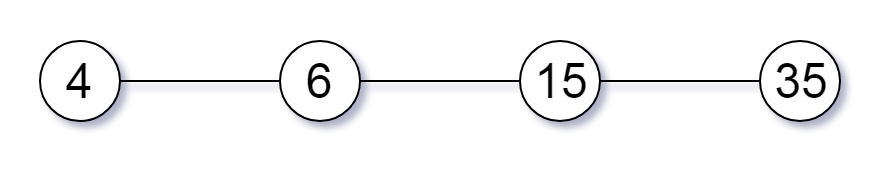Example 2:

Input: [20,50,9,63]
Output: 2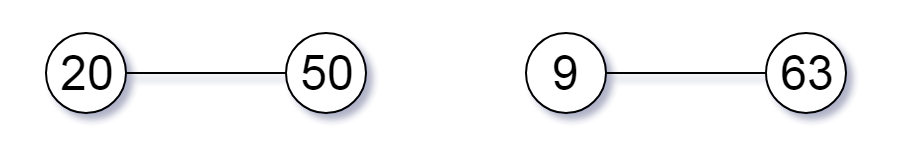Example 3:

Input: [2,3,6,7,4,12,21,39]
Output: 8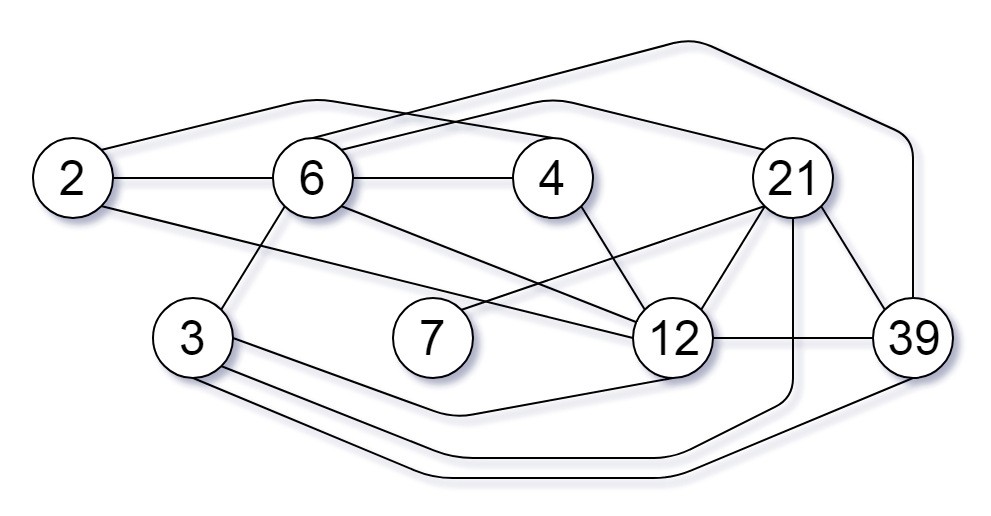Note:

1. 1 <= A.length <= 20000
2. 1 <= A[i] <= 100000

# Solution: Union Find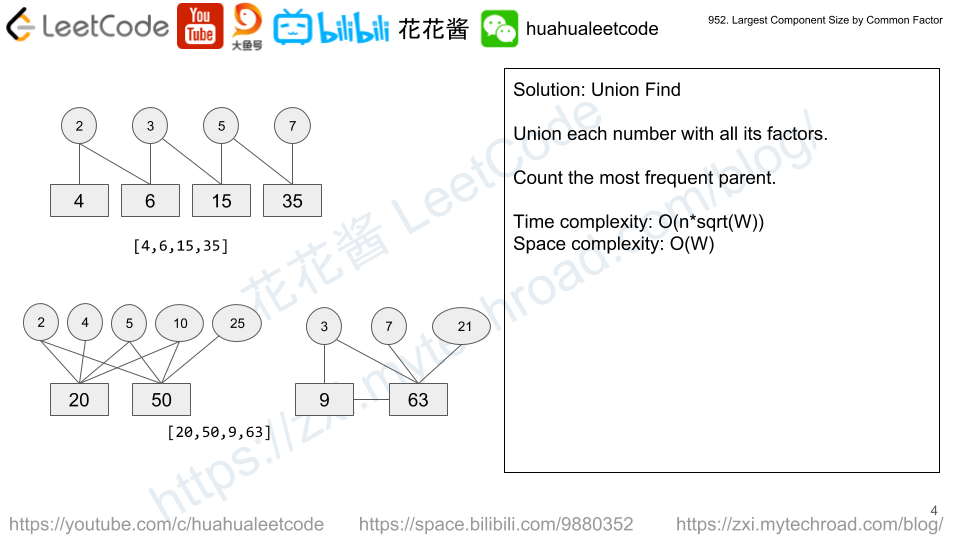For each number, union itself with all its factors.

E.g. 6, union(6,2), union(6,3)

Time complexity: $$O(\Sigma{sqrt(A[i])})$$

Space complexity: $$O(max(A))$$

# Problem

On a 2D plane, we place stones at some integer coordinate points.  Each coordinate point may have at most one stone.

Now, a move consists of removing a stone that shares a column or row with another stone on the grid.

What is the largest possible number of moves we can make?

Example 1:

Input: stones = [[0,0],[0,1],[1,0],[1,2],[2,1],[2,2]]
Output: 5


Example 2:

Input: stones = [[0,0],[0,2],[1,1],[2,0],[2,2]]
Output: 3


Example 3:

Input: stones = [[0,0]]
Output: 0


Note:

1. 1 <= stones.length <= 1000
2. 0 <= stones[i][j] < 10000

# Solution 2: Union Find

Find all connected components (islands)

Ans = # of stones – # of islands

# Related Problems

Mission News Theme by Compete Themes.